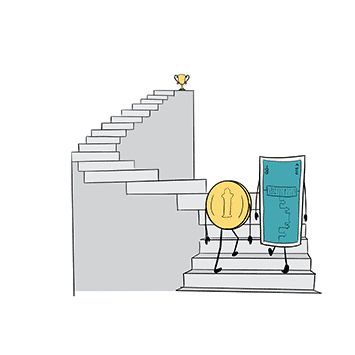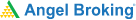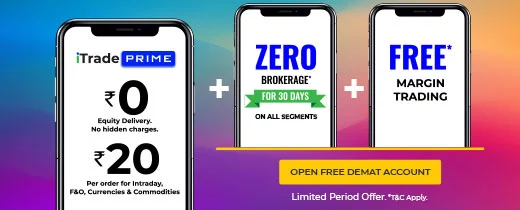# Glossary (20 terms to know about options and futures)

4.7## 1. Underlying asset

The asset on which the value of a derivative is based is known as the underlying asset. It can be anything from stocks and indexes to currencies and commodities.

## 2. Spot price

The spot price is the price at which an asset is being traded for in the spot (cash) market.

## 3. Call option

An option that gives you the right to buy an asset at a predetermined price and at a predetermined date is known as a call option.

## 4. Put option

An option that gives you the right to sell an asset at a predetermined price and on a predetermined date is known as a put option.

## 5. Bid price

The highest price that a buyer of a contract is willing to pay is known as the bid price.

The lowest price that a seller of a contract is willing to accept is known as the ask price.

## 7. Strike price

The strike price is the price at which you, as the owner of an option, get to either buy or sell the underlying asset on the predetermined date.

## 8. Lot size

A lot size is the minimum quantity of asset covered by a derivative contract. For instance, the lot size of a single futures or an options contract of HDFC Bank is 550 shares.

Also known as options premium, it is the price that the buyer of an options contract pays to the seller of the options contract.

## 10. Expiry

Also known as contract expiry, it is the date on which a derivative contract ceases to exist. If you don’t square off your open positions before the day of the expiry, you will either have to buy or sell the underlying asset according to the terms of your contract.

Learning & Earning is now super simple₹ 0 Equity Delivery

No Hidden Charges₹ 20 Per Order For Intraday

FAQ,Currencies & CommoditiesZERO Brokerage*

on ALL SegmentsFREE Margin

## 11. Long

The term long is used to denote the buying of either a futures contract or an options contract.

## 12. Short

The term short is used to denote the selling of either a futures contract or an options contract.

## 13. Intrinsic value

The intrinsic value is the difference between the spot price of the underlying asset and the strike price of the option contract of the underlying asset. The intrinsic value of a call option can be calculated by subtracting the strike price from the spot price, while the intrinsic value of a put option can be calculated by subtracting the spot price from the strike price.

## 14. In-the-money option

If the intrinsic value of an options contract, whether a call option or a put option, is a positive number, then the option is termed as an in-the-money option.

## 15. At-the-money option

An option whose strike price is equal to that of the underlying asset’s spot price is known as an at-the-money option. However, the chances of both the spot price and the strike price coinciding is slim. Therefore, an option whose strike price is closest to the spot price is also considered as an at-the-money option.

## 16. Out-of-the-money option

If the intrinsic value of an options contract, whether a call option or a put option, is zero, then the option is termed as an out-of-the-money option.

## 17. Open interest

The open interest is the total number of open contracts of a particular financial asset in the market.

## 18. Margin

The amount that you deposit with an exchange to either buy or sell a futures contract is known as the ‘margin.’ This margin money is only a percentage of the entire contract value and is liable to change according to the price movements of the underlying asset.

## 19. Margin call

When your initial margin money is not sufficient to cover your mark to market (MTM) losses, the stock exchange demands an additional margin deposit to nullify the risk of default. This demand for additional margin deposit is known as ‘margin call.’

## 20. Leverage

Leverage is the ability to control a contract with a large value by simply depositing a fraction of the total value. For instance, when you buy or sell a futures or an options contract with a large contract value by depositing a small margin or a premium amount, you’re said to have utilised leverage.

How would you rate this chapter?

25 Mar 2021, 01:05 AM

This is really helpful to clear your fundamentals. Strong fundamentals creates strong base. Thanks to team for working on this topic so beautifully.

Get Information Mindfulness!

Catch-up With Market

News in 60 Seconds.The perfect starter to begin and stay tuned with your learning journey anytime and anywhere.